# SciPy | Curve Fitting

Given a Dataset comprising of a group of points, find the best fit representing the Data.

We often have a dataset comprising of data following a general path, but each data has a standard deviation which makes them scattered across the line of best fit. We can get a single line using `curve-fit()` function.

Using SciPy :
Scipy is the scientific computing module of Python providing in-built functions on a lot of well-known Mathematical functions. The `scipy.optimize` package equips us with multiple optimization procedures. A detailed list of all functionalities of Optimize can be found on typing the following in the iPython console:

`help(scipy.optimize)`

Among the most used are Least-Square minimization, curve-fitting, minimization of multivariate scalar functions etc.

Curve Fitting Examples –

Input :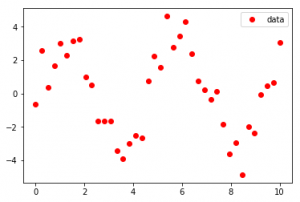Output :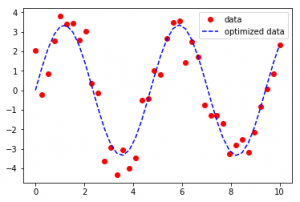Input :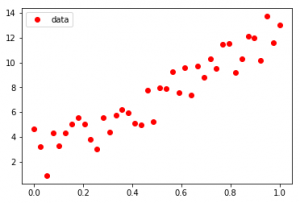Output :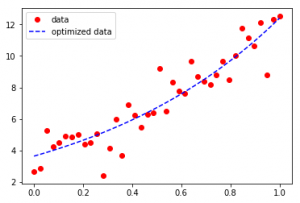As seen in the input, the Dataset seems to be scattered across a sine function in the first case and an exponential function in the second case, Curve-Fit gives legitimacy to the functions and determines the coefficients to provide the line of best fit.

Code showing the generation of the first example –

 `import` `numpy as np ` ` `  `# curve-fit() function imported from scipy ` `from` `scipy.optimize ``import` `curve_fit ` ` `  `from` `matplotlib ``import` `pyplot as plt ` ` `  `# numpy.linspace with the given arguments ` `# produce an array of 40 numbers between 0 ` `# and 10, both inclusive ` `x ``=` `np.linspace(``0``, ``10``, num ``=` `40``) ` ` `  ` `  `# y is another array which stores 3.45 times ` `# the sine of (values in x) * 1.334.  ` `# The random.normal() draws random sample  ` `# from normal (Gaussian) distribution to make ` `# them scatter across the base line ` `y ``=` `3.45` `*` `np.sin(``1.334` `*` `x) ``+` `np.random.normal(size ``=` `40``) ` ` `  `# Test function with coefficients as parameters ` `def` `test(x, a, b): ` `    ``return` `a ``*` `np.sin(b ``*` `x) ` ` `  `# curve_fit() function takes the test-function ` `# x-data and y-data as argument and returns  ` `# the coefficients a and b in param and ` `# the estimated covariance of param in param_cov ` `param, param_cov ``=` `curve_fit(test, x, y) ` ` `  ` `  `print``(``"Sine funcion coefficients:"``) ` `print``(param) ` `print``(``"Covariance of coefficients:"``) ` `print``(param_cov) ` ` `  `# ans stores the new y-data according to  ` `# the coefficients given by curve-fit() function ` `ans ``=` `(param[``0``]``*``(np.sin(param[``1``]``*``x))) ` ` `  `'''Below 4 lines can be un-commented for plotting results  ` `using matplotlib as shown in the first example. '''` ` `  `# plt.plot(x, y, 'o', color ='red', label ="data") ` `# plt.plot(x, ans, '--', color ='blue', label ="optimized data") ` `# plt.legend() ` `# plt.show() `

Output:

```Sine function coefficients:
[ 3.66474998  1.32876756]
Covariance of coefficients:
[[  5.43766857e-02  -3.69114170e-05]
[ -3.69114170e-05   1.02824503e-04]]
```

Second example can be achieved by using the numpy exponential function shown as follows:

 `x ``=` `np.linspace(``0``, ``1``, num ``=` `40``) ` ` `  `y ``=` `3.45` `*` `np.exp(``1.334` `*` `x) ``+` `np.random.normal(size ``=` `40``) ` ` `  `def` `test(x, a, b): ` `    ``return` `a``*``np.exp(b``*``x) ` ` `  `param, param_cov ``=` `curve_fit(test, x, y) `

However, if the coefficinets are too large, the curve flattens and fails to provide the best fit. The following code explains this fact:

 `import` `numpy as np ` `from` `scipy.optimize ``import` `curve_fit ` ` `  `from` `matplotlib ``import` `pyplot as plt ` ` `  `x ``=` `np.linspace(``0``, ``10``, num ``=` `40``) ` ` `  `# The coefficients are much bigger. ` `y ``=` `10.45` `*` `np.sin(``5.334` `*` `x) ``+` `np.random.normal(size ``=` `40``) ` ` `  `def` `test(x, a, b): ` `    ``return` `a ``*` `np.sin(b ``*` `x) ` ` `  `param, param_cov ``=` `curve_fit(test, x, y) ` ` `  `print``(``"Sine funcion coefficients:"``) ` `print``(param) ` `print``(``"Covariance of coefficients:"``) ` `print``(param_cov) ` ` `  `ans ``=` `(param[``0``]``*``(np.sin(param[``1``]``*``x))) ` ` `  `plt.plot(x, y, ``'o'``, color ``=``'red'``, label ``=``"data"``) ` `plt.plot(x, ans, ``'--'``, color ``=``'blue'``, label ``=``"optimized data"``) ` `plt.legend() ` `plt.show() `

Output:

```Sine funcion coefficients:
[ 0.70867169  0.7346216 ]
Covariance of coefficients:
[[ 2.87320136 -0.05245869]
[-0.05245869  0.14094361]]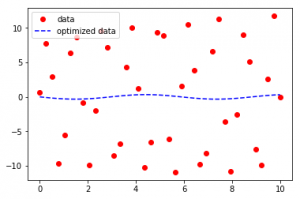```

The blue dotted line is undoubtedly the line with best-optimized distances from all points of the dataset, but it fails to provide a sine function with the best fit.

Curve Fitting should not be confused with Regression. They both involve approximating data with functions. But the goal of Curve-fitting is to get the values for a Dataset through which a given set of explanatory variables can actually depict another variable. Regression is a special case of curve fitting but here you just don’t need a curve which fits the training data in the best possible way(which may lead to overfitting) but a model which is able to generalize the learning and thus predict new points efficiently.

Attention geek! Strengthen your foundations with the Python Programming Foundation Course and learn the basics.

To begin with, your interview preparations Enhance your Data Structures concepts with the Python DS Course.

My Personal Notes arrow_drop_upCheck out this Author's contributed articles.

If you like GeeksforGeeks and would like to contribute, you can also write an article using contribute.geeksforgeeks.org or mail your article to contribute@geeksforgeeks.org. See your article appearing on the GeeksforGeeks main page and help other Geeks.

Please Improve this article if you find anything incorrect by clicking on the "Improve Article" button below.